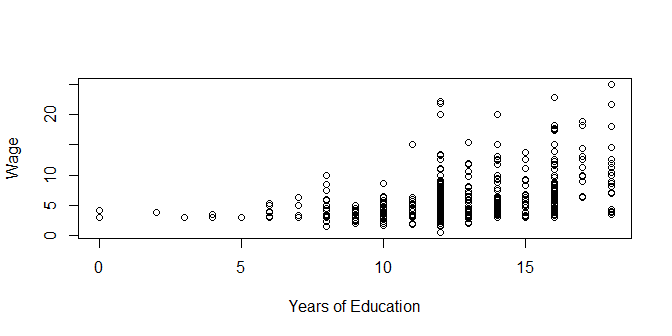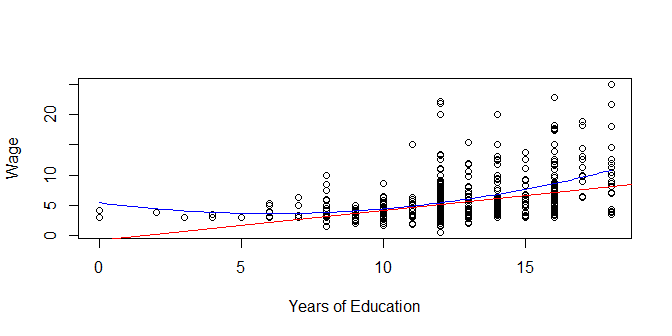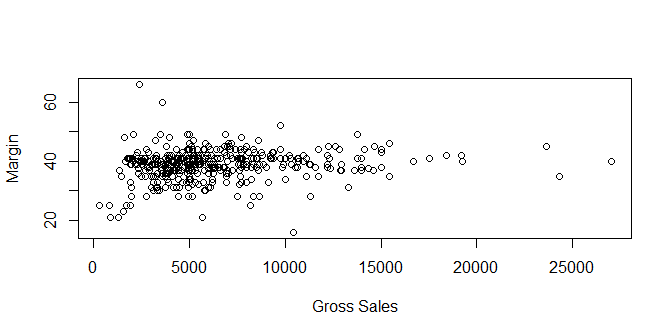# Lecture 9: Relationships Between Variables, Part 1

## Recap

• Summary statistics are ways of describing the distribution of a variable
• We can also just look at the variable directly
• Understanding a variable’s distribution is important if we want to use it

## This week

• We aren’t just interested in looking at variables by themselves!
• We want to know how variables can be related to each other
• When `X` is high, would we expect `Y` to also be high, or be low?
• How are variables correlated?
• How does one variable explain another?
• How does one variable cause another? (later!)

## An Example: Dependence

``table(wage1\$numdep,wage1\$smsa,dnn=c('Num. Dependents','Lives in Metropolitan Area'))``
``````##                Lives in Metropolitan Area
## Num. Dependents   0   1
##               0  60 192
##               1  27  78
##               2  38  61
##               3  13  32
##               4   3  13
##               5   3   4
##               6   2   0``````

## An Example: Dependence

• What are we looking for here?
• For dependence, simply see if the distribution of one variable changes for the different values of the other.
• Does the distribution of Number of Dependents differ based on your SMSA status?
``prop.table(table(wage1\$numdep,wage1\$smsa,dnn=c('Num. Dependents','Lives in Metropolitan Area')),margin=2)``
``````##                Lives in Metropolitan Area
## Num. Dependents          0          1
##               0 0.41095890 0.50526316
##               1 0.18493151 0.20526316
##               2 0.26027397 0.16052632
##               3 0.08904110 0.08421053
##               4 0.02054795 0.03421053
##               5 0.02054795 0.01052632
##               6 0.01369863 0.00000000``````

## An Example: Dependence

• Does the distribution of SMSA differ based on your Number of Dependents Status?
``prop.table(table(wage1\$numdep,wage1\$smsa,dnn=c('Number of Dependents','Lives in Metropolitan Area')),margin=1)``
``````##                     Lives in Metropolitan Area
## Number of Dependents         0         1
##                    0 0.2380952 0.7619048
##                    1 0.2571429 0.7428571
##                    2 0.3838384 0.6161616
##                    3 0.2888889 0.7111111
##                    4 0.1875000 0.8125000
##                    5 0.4285714 0.5714286
##                    6 1.0000000 0.0000000``````
• Looks like it!
• What do these two results mean?

## An Example: Correlation

• We are interested in whether two variables tend to move together (positive correlation) or move apart (negative correlation)
• One basic way to do this is to see whether values tend to be high together
• One way to check in dplyr is to use `group_by()` to organize the data into groups
• Then `summarize()` the data within those groups
``````wage1 %>%
group_by(smsa) %>%
summarize(numdep=mean(numdep))``````
``````## # A tibble: 2 x 2
##    smsa numdep
##   <dbl>  <dbl>
## 1     0  1.24
## 2     1  0.968``````
• When `smsa` is high, `numdep` tends to be low - negative correlation!

## An Example: Correlation

• There’s also a summary statistic we can calculate called correlation, this is typically what we mean by “correlation”
• Ranges from -1 (perfect negative correlation) to 1 (perfect positive correlation)
• Basically “a one-standard deviation increase in `X` is associated with a correlation-standard-deviation increase in `Y`
``cor(wage1\$numdep,wage1\$smsa)``
``##  -0.09636769``
``cor(wage1\$smsa,wage1\$numdep)``
``##  -0.09636769``

## An Example: Explanation

Let’s go back to those different means:

``````## # A tibble: 2 x 2
##    smsa numdep
##   <dbl>  <dbl>
## 1     0  1.24
## 2     1  0.968``````
• Explanation would be saying that, based on this, if you’re in an SMSA, I predict that you have 0.9684211 dependents, and if you’re not, you have 1.239726 dependents
• If you are in an SMSA and have 2 dependents, then 0.9684211 of those dependents are explained by SMSA and 2 - 0.9684211 = 1.0315789 of them are unexplained by SMSA

## Coding Recap

• `table(df\$var1,df\$var2)` to look at two variables together
• `prop.table(table(df\$var1,df\$var2))` for the proportion in each cell
• `prop.table(table(df\$var1,df\$var2),margin=2)` to get proportions within each column
• `prop.table(table(df\$var1,df\$var2),margin=1)` to get proportions within each row
• `df %>% group_by(var1) %>% summarize(mean(var2))` to get mean of var2 for each value of var1
• `cor(df\$var1,df\$var2)` to calculate correlation

## Graphing Relationships

• Relationships between variables can be easier to see graphically
• And graphs are extremely important to understanding relationships and the “shape” of those relationships

## Wage and Education

• Let’s use `plot(xvar,yvar)` with two variables
``plot(wage1\$educ,wage1\$wage,xlab="Years of Education",ylab="Wage")``• As we look at different values of `educ`, what changes about the values of `wage` we see?

## Graphing Relationships

• Try to picture the shape of the data
• Should this be a straight line? A curved line? Positively sloped? Negatively?
``````plot(wage1\$educ,wage1\$wage,xlab="Years of Education",ylab="Wage")
abline(-.9,.5,col='red')## Graphing Relationships

• `plot(xvar,yvar)` is extremely powerful, and will show you relationships at a glance
• The previous graph showed a clear positive relationship, and indeed `cor(wage1\$wage,wage1\$educ)` = 0.4059033
• Further, we don’t only see a positive relationship, but we have some sense of how positive it is, what it looks like roughly
• Let’s look at some more

## Graphing Relationships

• Let’s compare clothing sales volume vs. profit margin for men’s clothing firms
``````library(Ecdat)
data(Clothing)
plot(Clothing\$sales,Clothing\$margin,xlab="Gross Sales",ylab="Margin")``````• No clear up-or-down relationship (although the correlation is 0.1373499!) but clearly the variance is higher for low sales

## Graphing Relationships

• Comparing Singapore diamond prices vs. carats
``````library(Ecdat)
data(Diamond)
plot(Diamond\$carat,Diamond\$price,xlab="Number of Carats",ylab="Price")``````